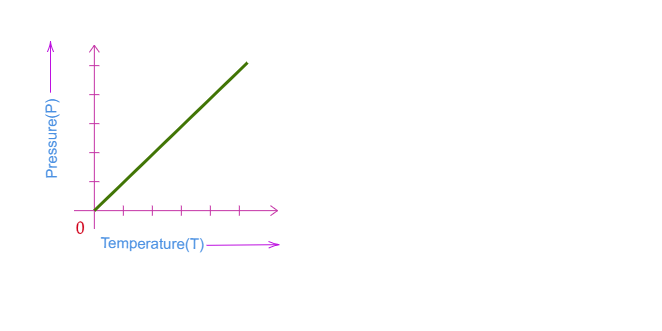# Explain Gay Lussac's law in detail.

Gay-Lussac's Pressure-Temperature law:

According to Gay-Lussac's Pressure-Temperature law, when the mass of a gas is fixed and the volume is constant then the exerted pressure by a gas is directly proportional to the temperature of the gas.

$P\propto T$

Or $P=kT$

Or $\frac{P}{T}=k$

where:

$P\rightarrow$pressure exerted by the gas

$T\rightarrow$ absolute temperature of the body

$k\rightarrow$ constant

This relationship between the exerted pressure and temperature of gas with constant mass and volume can be shown on the graph as below:Updated on: 10-Oct-2022

48 Views